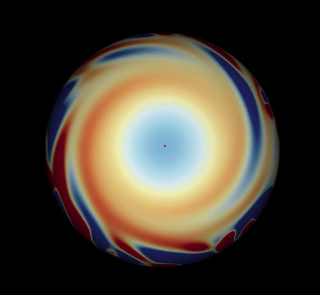## Surface flux transport

### Description

This applied project will explore the evolution of magnetic flux patterns on the surface of the Sun, using the surface flux transport (SFT) model first proposed by R.B. Leighton in the 1960s. New magnetic flux emerges rapidly in concentrated "active regions" (sunspots), before undergoing a gradual spreading out and decay. In addition, the decaying patterns are carried around by large-scale surface motions. Mathematically, we have an advection-diffusion equation for a passive scalar field, which occurs in other contexts too.An SFT simulation of the Sun's surface, looking down from the north pole. Red represents positive magnetic flux (i.e., out of the Sun), and blue negative.

We will begin by looking at the underlying advection-diffusion equation (a PDE) and its physical motivation. There are some useful exact solutions in simplified cases - for example, if we neglect the poleward "meridional" flow, or if we look at a local region where the curvature of the solar surface can be neglected. But for realistic solutions you will need to write a numerical Python code to solve the equation. No prior experience of solving PDEs numerically will be assumed, so the project would be a nice introduction to this topic.

### Prerequisites & corequisites

Analysis in Many Variables II, and some familiarity with numerical computations in Python from either Numerical Analysis II or Mathematical Modelling II. Although the project involves magnetic fields, there will be little or no overlap with Special Relativity & Electromagnetism II.

There are no required corequisites, but the project would nicely complement Fluid Mechanics III and/or Mathematical Biology III.

### Resources [online]

To get a flavour of the physical model, you could try Neil Sheeley's article Surface Evolution of the Sun's Magnetic Field: A Historical Review of the Flux-Transport Mechanism. Or Chapter 2 of this review. There are additional review papers by Jiang et al. and Wang, listing many other references. Leighton's original paper is also an accessible read. For the numerical solution of partial differential equations, you will find introductory material in many textbooks on Numerical Analysis. For a detailed but readable introduction to methods for fluids, including advection-diffusion, see A First Course in Computational Fluid Dynamics (Aref & Balachandar). Ingo Baumann's PhD thesis describes a neat method for solving the SFT equation using spherical harmonics.

email: A Yeates